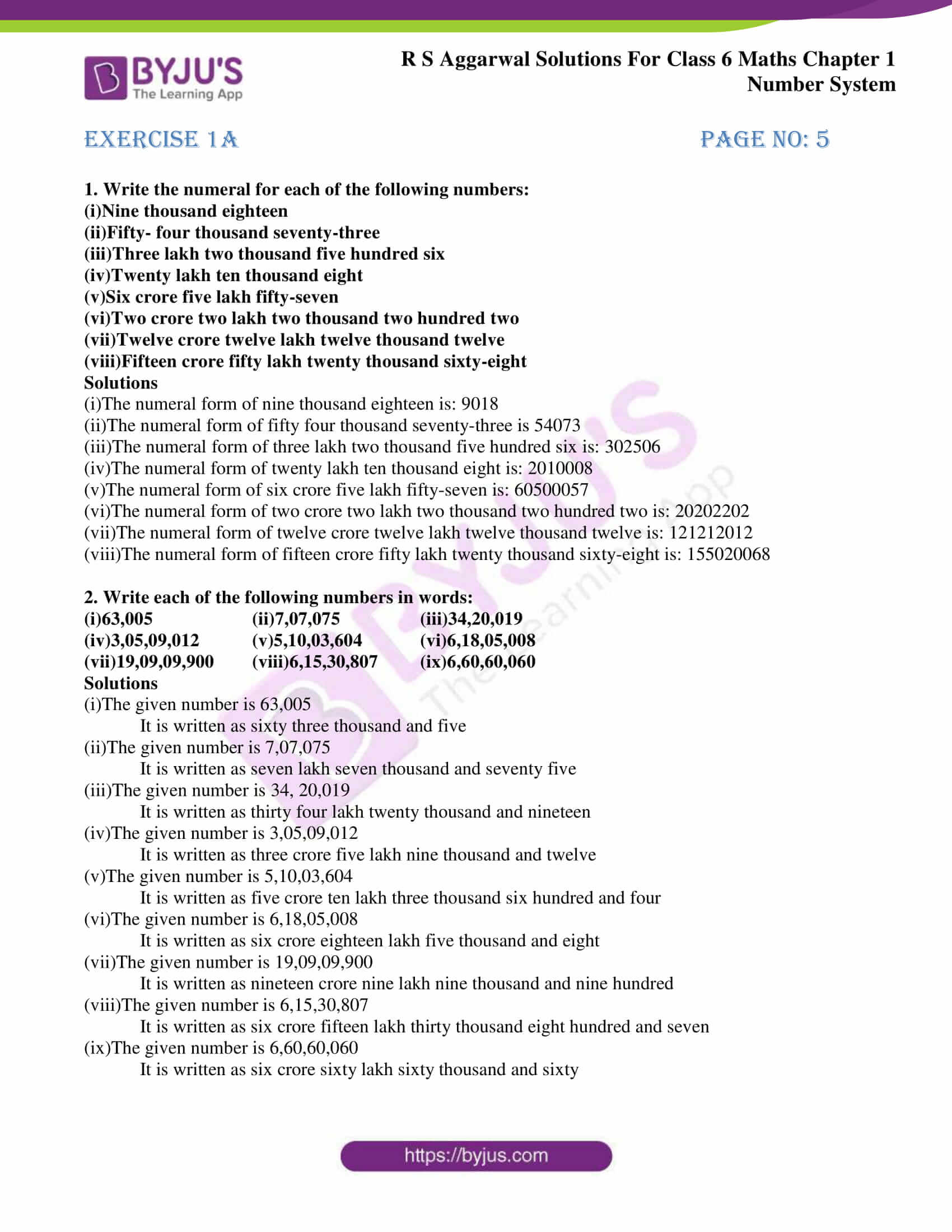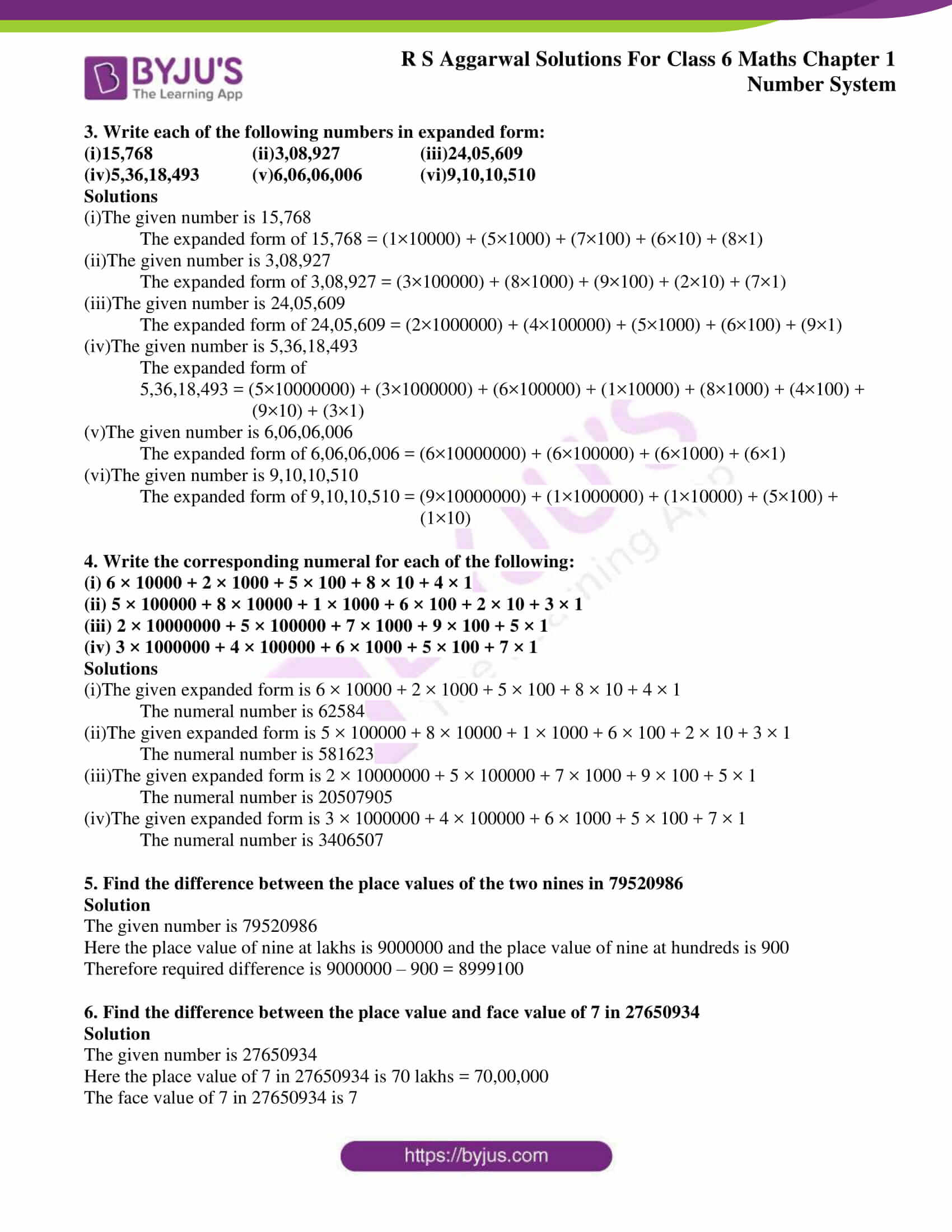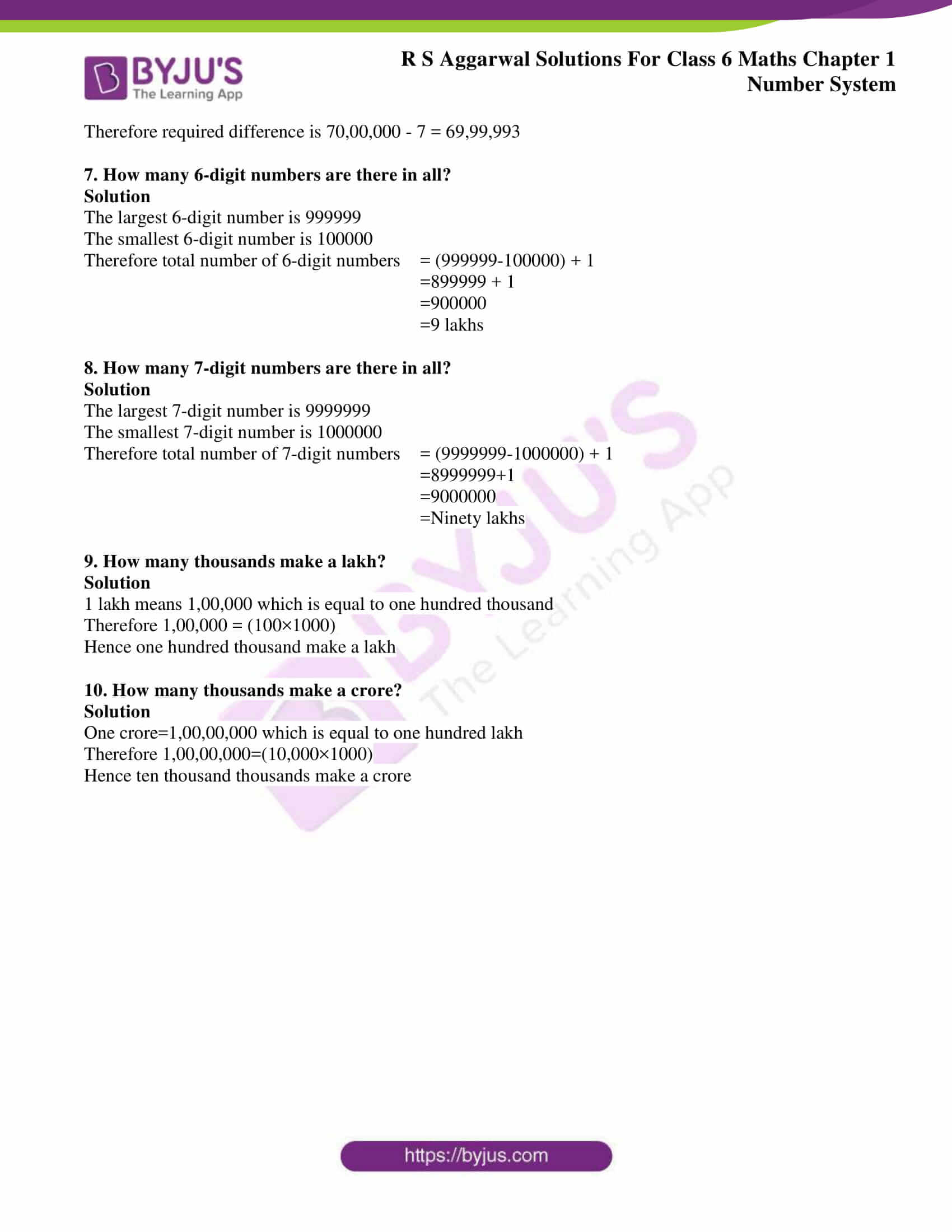# RS Aggarwal Solutions for Class 6 Chapter 1 Number System Exercise 1A

Students can download the RS Aggarwal Solutions for Class 6 Chapter 1, Number System Exercise 1A PDF from the link which is available here. The subject experts at BYJU’S have solved the questions present in RS Aggarwal textbook Chapter 1 Exercise 1A. These solutions help students in understanding the concepts without any difficulty. These problems are mainly based on the international system of numeration.

To increase the level of confidence and to score high marks, students are advised to practice the RS Aggarwal Solutions for Class 6 Maths, which plays a vital role in their examination. RS Aggarwal textbook for Class 6 Chapter 1 Number system Exercise 1A PDF are provided here.

## Download Pdf of RS Aggarwal Solutions for Class 6 Chapter 1 Number System Exercise 1A### Access answers to Maths RS Aggarwal Solutions for Class 6 Chapter 1

1. Write the numeral for each of the following numbers:

(i)Nine thousand eighteen

(ii)Fifty- four thousand seventy-three

(iii)Three lakh two thousand five hundred six

(iv)Twenty lakh ten thousand eight

(v)Six crore five lakh fifty-seven

(vi)Two crore two lakh two thousand two hundred two

(vii)Twelve crore twelve lakh twelve thousand twelve

(viii)Fifteen crore fifty lakh twenty thousand sixty-eight

Solutions

(i)The numeral form of nine thousand eighteen is: 9018

(ii)The numeral form of fifty four thousand seventy-three is 54073

(iii)The numeral form of three lakh two thousand five hundred six is: 302506

(iv)The numeral form of twenty lakh ten thousand eight is: 2010008

(v)The numeral form of six crore five lakh fifty-seven is: 60500057

(vi)The numeral form of two crore two lakh two thousand two hundred two is: 20202202

(vii)The numeral form of twelve crore twelve lakh twelve thousand twelve is: 121212012

(viii)The numeral form of fifteen crore fifty lakh twenty thousand sixty-eight is: 155020068

2. Write each of the following numbers in words:

(i)63,005 (ii)7,07,075 (iii)34,20,019

(iv)3,05,09,012 (v)5,10,03,604 (vi)6,18,05,008

(vii)19,09,09,900 (viii)6,15,30,807 (ix)6,60,60,060

Solutions

(i)The given number is 63,005

It is written as sixty three thousand and five

(ii)The given number is 7,07,075

It is written as seven lakh seven thousand and seventy five

(iii)The given number is 34, 20,019

It is written as thirty four lakh twenty thousand and nineteen

(iv)The given number is 3,05,09,012

It is written as three crore five lakh nine thousand and twelve

(v)The given number is 5,10,03,604

It is written as five crore ten lakh three thousand six hundred and four

(vi)The given number is 6,18,05,008

It is written as six crore eighteen lakh five thousand and eight

(vii)The given number is 19,09,09,900

It is written as nineteen crore nine lakh nine thousand and nine hundred

(viii)The given number is 6,15,30,807

It is written as six crore fifteen lakh thirty thousand eight hundred and seven

(ix)The given number is 6,60,60,060

It is written as six crore sixty lakh sixty thousand and sixty

3. Write each of the following numbers in expanded form:

(i)15,768 (ii)3,08,927 (iii)24,05,609

(iv)5,36,18,493 (v)6,06,06,006 (vi)9,10,10,510

Solutions

(i)The given number is 15,768

The expanded form of 15,768 = (1×10000) + (5×1000) + (7×100) + (6×10) + (8×1)

(ii)The given number is 3,08,927

The expanded form of 3,08,927 = (3×100000) + (8×1000) + (9×100) + (2×10) + (7×1)

(iii)The given number is 24,05,609

The expanded form of 24,05,609 = (2×1000000) + (4×100000) + (5×1000) + (6×100) + (9×1)

(iv)The given number is 5,36,18,493

The expanded form of

5,36,18,493 = (5×10000000) + (3×1000000) + (6×100000) + (1×10000) + (8×1000) + (4×100) +

(9×10) + (3×1)

(v)The given number is 6,06,06,006

The expanded form of 6,06,06,006 = (6×10000000) + (6×100000) + (6×1000) + (6×1)

(vi)The given number is 9,10,10,510

The expanded form of 9,10,10,510 = (9×10000000) + (1×1000000) + (1×10000) + (5×100) +

(1×10)

4. Write the corresponding numeral for each of the following:

(i) 6 × 10000 + 2 × 1000 + 5 × 100 + 8 × 10 + 4 × 1

(ii) 5 × 100000 + 8 × 10000 + 1 × 1000 + 6 × 100 + 2 × 10 + 3 × 1

(iii) 2 × 10000000 + 5 × 100000 + 7 × 1000 + 9 × 100 + 5 × 1

(iv) 3 × 1000000 + 4 × 100000 + 6 × 1000 + 5 × 100 + 7 × 1

Solutions

(i)The given expanded form is 6 × 10000 + 2 × 1000 + 5 × 100 + 8 × 10 + 4 × 1

The numeral number is 62584

(ii)The given expanded form is 5 × 100000 + 8 × 10000 + 1 × 1000 + 6 × 100 + 2 × 10 + 3 × 1

The numeral number is 581623

(iii)The given expanded form is 2 × 10000000 + 5 × 100000 + 7 × 1000 + 9 × 100 + 5 × 1

The numeral number is 20507905

(iv)The given expanded form is 3 × 1000000 + 4 × 100000 + 6 × 1000 + 5 × 100 + 7 × 1

The numeral number is 3406507

5. Find the difference between the place values of the two nines in 79520986

Solution

The given number is 79520986

Here the place value of nine at lakhs is 9000000 and the place value of nine at hundreds is 900

Therefore required difference is 9000000 – 900 = 8999100

6. Find the difference between the place value and face value of 7 in 27650934

Solution

The given number is 27650934

Here the place value of 7 in 27650934 is 70 lakhs = 70,00,000

The face value of 7 in 27650934 is 7

Therefore required difference is 70,00,000 – 7 = 69,99,993

7. How many 6-digit numbers are there in all?

Solution

The largest 6-digit number is 999999

The smallest 6-digit number is 100000

Therefore total number of 6-digit numbers = (999999-100000) + 1

=899999 + 1

=900000

=9 lakhs

8. How many 7-digit numbers are there in all?

Solution

The largest 7-digit number is 9999999

The smallest 7-digit number is 1000000

Therefore total number of 7-digit numbers = (9999999-1000000) + 1

=8999999+1

=9000000

=Ninety lakhs

9. How many thousands make a lakh?

Solution

1 lakh means 1,00,000 which is equal to one hundred thousand

Therefore 1,00,000 = (100×1000)

Hence one hundred thousand make a lakh

10. How many thousands make a crore?

Solution

One crore=1,00,00,000 which is equal to one hundred lakh

Therefore 1,00,00,000=(10,000×1000)

Hence ten thousand thousands make a crore

## Number System Exercise 1A

RS Aggarwal Solutions for Exercise 1A deals with the basic concept of Number System. By regular practice, students gain a better idea about Number system. Some of the primary topics mentioned in other exercises are as follows

• Comparison of numbers
• Word problem of number operations
• Estimation
• Estimating the products
• Estimating the quotients
• Roman numerals

Students can use the RS Aggarwal solutions which contains solutions for every question mentioned in the textbook to score high marks in Maths. The students can download PDF of solutions to obtain a better academic score.